U.S. Department of Transportation
1200 New Jersey Avenue, SE
Washington, DC 20590
202-366-4000

Federal Highway Administration Research and Technology
Coordinating, Developing, and Delivering Highway Transportation InnovationsThis report is an archived publication and may contain dated technical, contact, and link information
 Federal Highway Administration > Publications > Research Publications > 04046 > Appg.Cfm Evaluation of Procedures for Quality Assurance Specifications
 Publication Number: FHWA-HRT-04-046 Date: October 2004

# Appendix G: Bias Histograms From The Simulation Program

The plots in appendix G illustrate the distributions of sample means for a number of different combinations of population PD, sample size, and skewness coefficients. These figures are an expansion of those shown and discussed in chapter 6.

Figure 114 shows how sample size can affect the variability of the PD estimates. This figure shows the complete program output for a symmetrical population (i.e., skewness coefficient = 0) that actually has 10 PD and for which the sample size is n = 5. The results of the nine divisions for PDL/PDU are clearly indicated. The BIAS/SE results indicate the average bias and the standard error (measure of variability of the PD estimates), respectively. These terms are discussed and explained in detail in chapter 6. The bias values are relatively closely distributed about zero, indicating that the method for estimating PD (and hence PWL) is not biased for a symmetrical distribution. This is to be expected since the PD estimating method assumes a symmetrical normal distribution.

Figure 115 shows portions of the program output for the same population, but with sample sizes of n = 3 and n = 10. Figures 116 and 117, and 118 and 119 show similar plots for populations with skewness coefficients = 1.0 and 2.0, respectively. The remaining figures illustrate other comparisons of the bias results. Figures 120 and 121 show the bias values histograms for sampling with the same sample size of n = 5 from symmetrical populations with different PD values (i.e., PD = 30 and PD = 50, respectively). Figures 122 and 123 show similar results for a sample size of n = 10. Figure 124 shows the bias values histograms for the same sample size of n = 5 and the same PD value = 50, but for populations with skewness coefficients = 0.0, 1.0, 2.0, and 3.0. Figures 125 through 127 show the complete program outputs for populations with a skewness coefficient = 3.0, a sample size of n = 5, and PD values = 10, 30, and 50. It is unlikely that populations with this degree of skewness will be found in practice.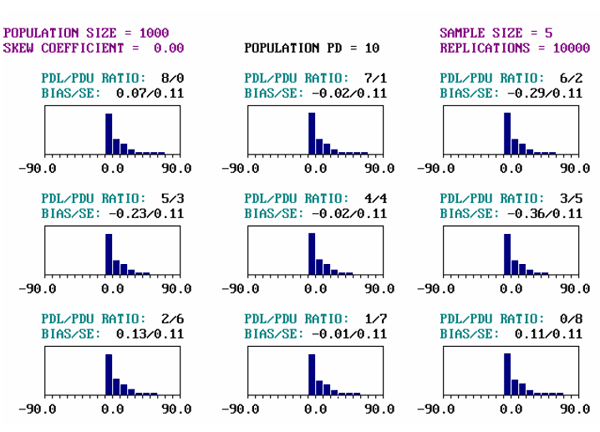Figure 114. Sample output screen for a population with PD = 10, skewness coefficient = 0.00, and sample size = 5.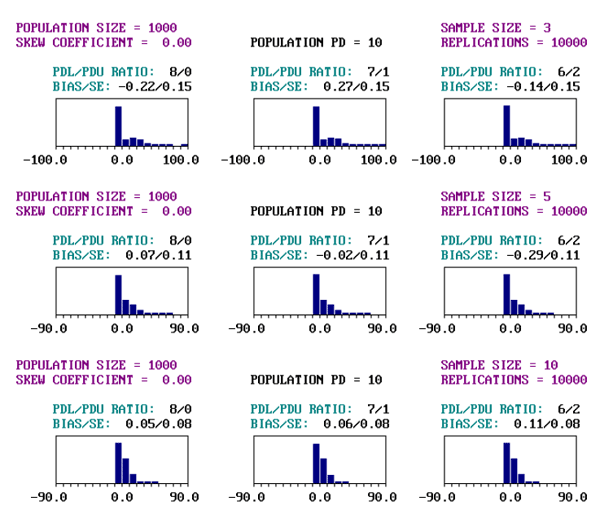Figure 115. Portions of output screens for PD = 10, skewness coefficient = 0.00, and sample sizes = 3, 5, and 10.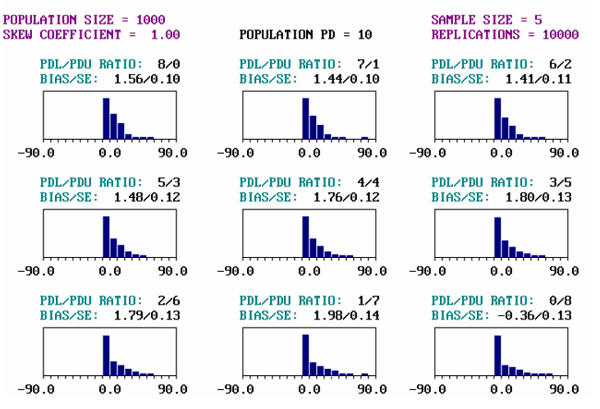Figure 116. Sample output screen for a population with PD = 10, skewness coefficient = 1.00, and sample size = 5.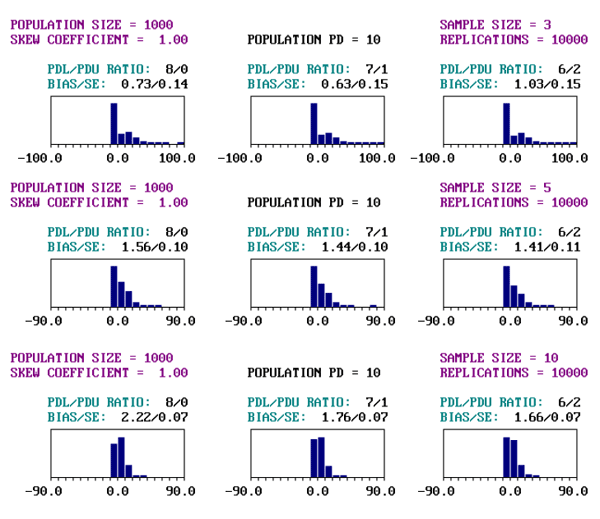Figure 117. Portions of output screens for PD = 10, skewness coefficient = 1.00, and sample sizes = 3, 5, and 10.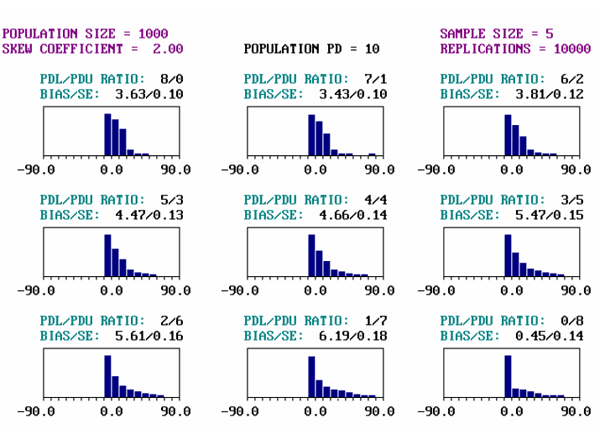Figure 118. Sample output screen for a population with PD = 10, skewness coefficient = 2.00, and sample size = 5.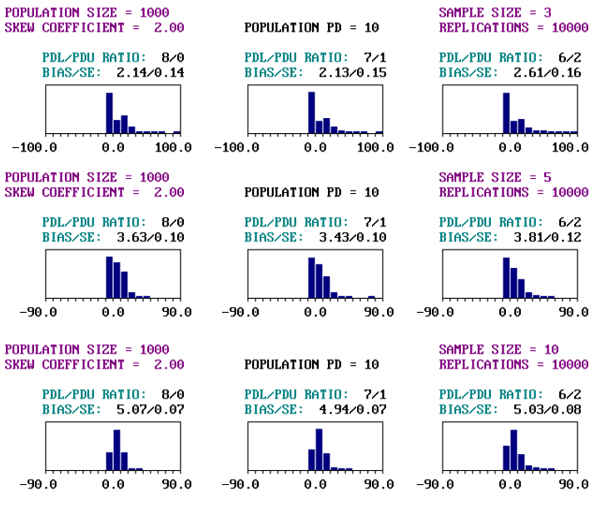Figure 119. Portions of output screens for PD = 10, skewness coefficient = 2.00, and sample sizes = 3, 5, and 10.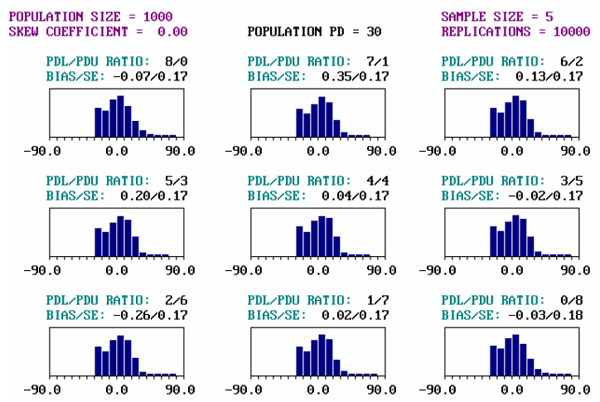Figure 120. Sample output screen for a population with PD = 30, skewness coefficient = 0.00, and sample size = 5.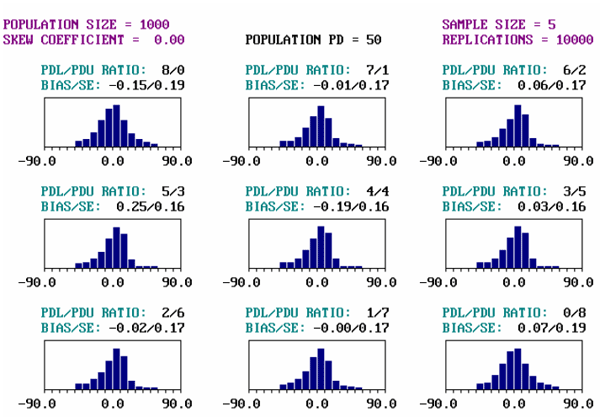Figure 121. Sample output screen for a population with PD = 50, skewness coefficient = 0.00, and sample size = 5.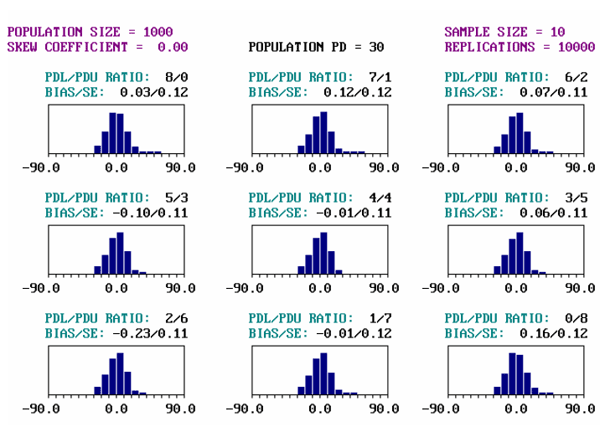Figure 122. Sample output screen for a population with PD = 30, skewness coefficient = 0.00, and sample size = 10.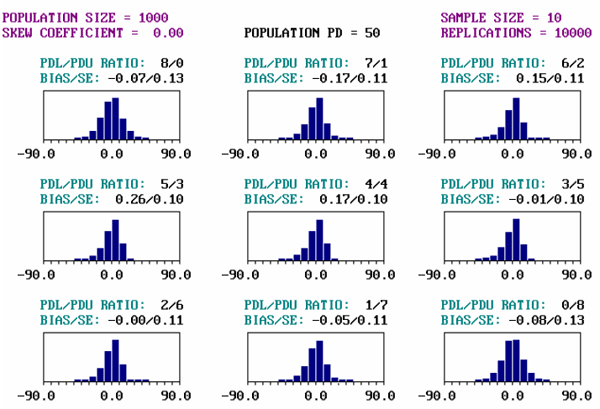Figure 123. Sample output screen for a population with PD = 50, skewness coefficient = 0.00, and sample size = 10.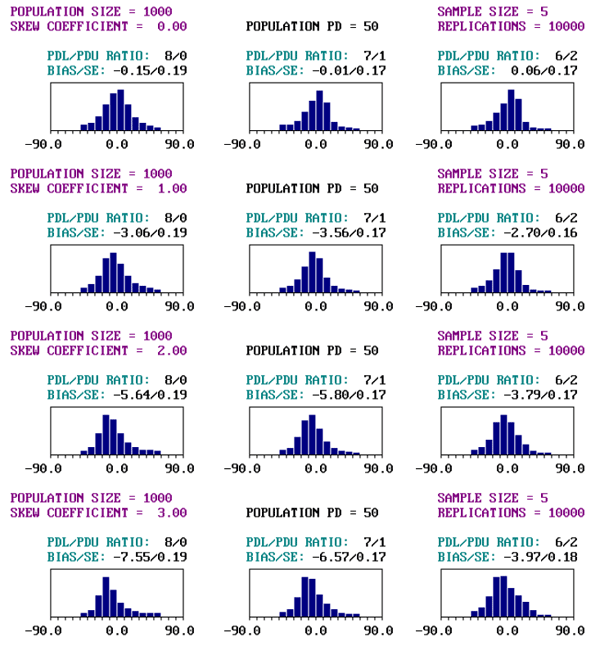Figure 124. Portions of output screens for PD = 50, sample size = 5, and skewness coefficients = 0.00, 1.00, 2.00, and 3.00.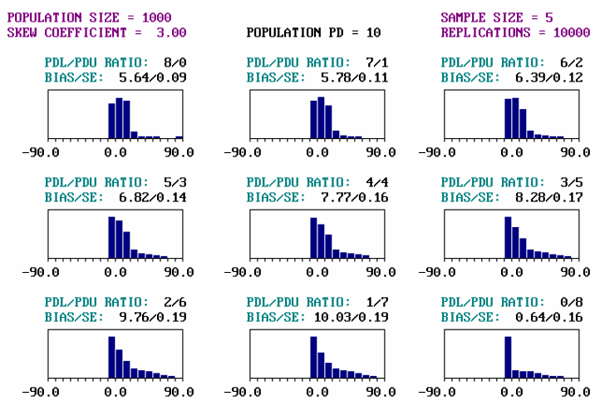Figure 125. Sample output screen for a population with PD = 10, skewness coefficient = 3.00, and sample size = 5.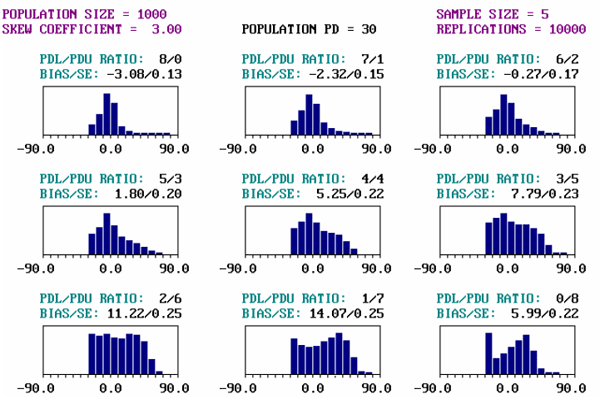Figure 126. Sample output screen for a population with PD = 30, skewness coefficient = 3.00, and sample size = 5.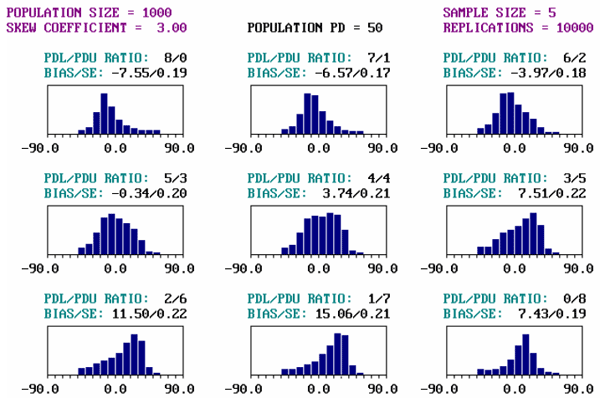Figure 127. Sample output screen for a population with PD = 50, skewness coefficient = 3.00, and sample size = 5.